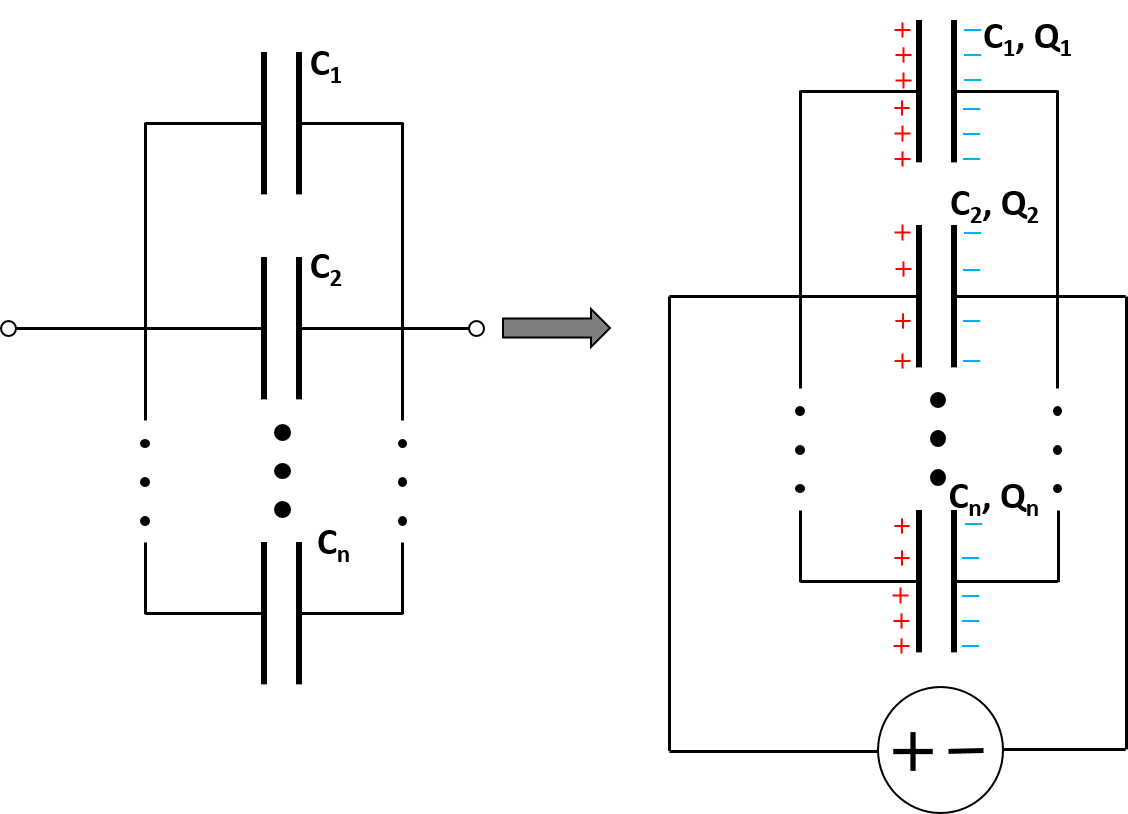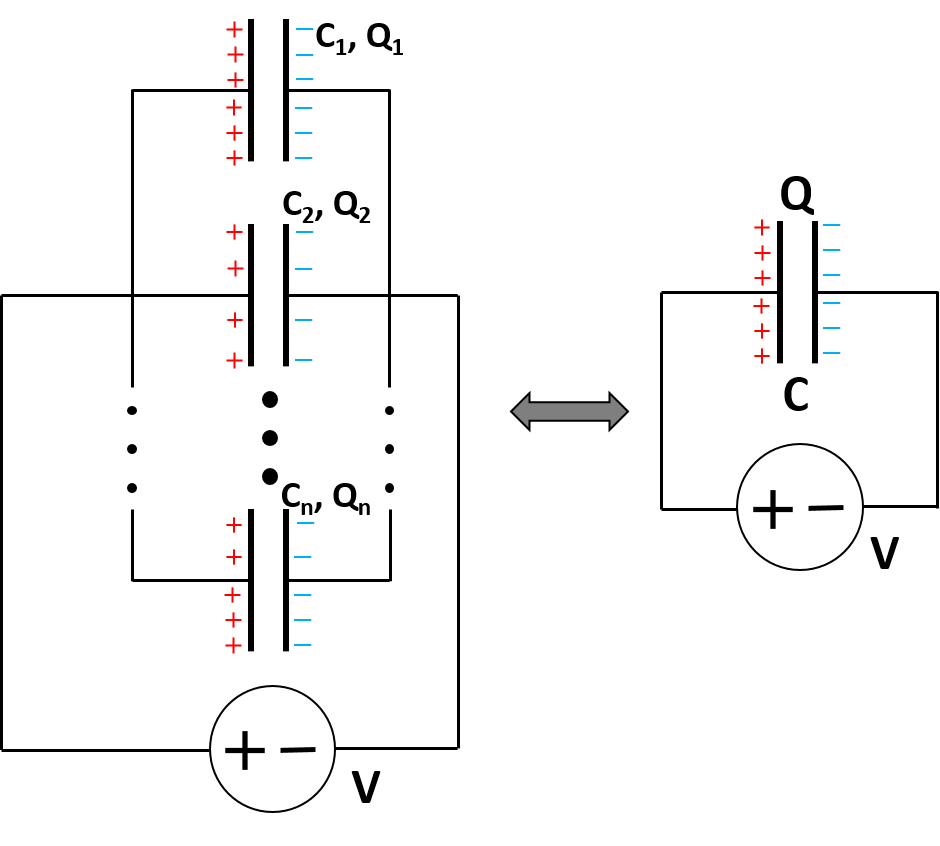# Parallel Capacitor Calculator

Created by Wojciech Sas, PhD candidate
Reviewed by Bogna Szyk and Jack Bowater
Last updated: Feb 24, 2022

This parallel capacitor calculator allows you to estimate the resulting capacitance in a circuit. You can simulate the arrangement of up to 10 separate capacitors in parallel. Additionally, we also provide the formula for parallel capacitors, as well as an explanation of where it comes from. We also provide you with an explanation into the differences between capacitors in series and in parallel, and how they corresponds to the formulas for resistors.

A capacitor is one of the most important electronic components. It acts as a place where some electric charge can be stored. Capacitors may be combined both in series and in parallel. The question is: What is the resulting capacitance for a circuit composed entirely of capacitors in parallel? Let's work it out together, using parallel plate capacitors as an example!When capacitors are arranged in parallel in a system with voltage source V, the voltages over each element is the same, and equal to the source capacitor:

V₁ = V₂ = ... = V.

The general formula for the charge, Qi, stored in capacitor, Ci, is:

 Qi = Vi * Ci 

If we want to replace all the elements with the substitutionary capacitance, C, we need to realise that the overall charge, Q, is the sum of all charges:

Q = Q₁ + Q₂ + ...,

which can also be written as:

V * C = V * C₁ + V * C₂ + ....Dividing both sides by V, we obtain the formula for the output capacity:

C = C₁ + C₂ + ....

As you can see, the capacitors in parallel formula is exactly the same as that for series resistors, which is simply the sum of all the individual components. It turns out that the equation for capacitors in series resembles the one for parallel resistors as well as parallel inductors.

In general, if we want to construct a system with higher capacitance, we should arrange the capacitors in parallel. On the other hand, if the capacitors are in series, the resulting capacity is lower than any of the individual components.

## How to use the parallel capacitor calculator?

Let's arrange a few capacitors in parallel and find the resulting capacitance. The starting set consists of the following capacitors: C₁ = 30 mF, C₂ = 500 µF, C₃ = 6 mF, C₄ = 750 µF.

1. To make our life easier, convert the units of capacitance so they are the same, e.g. mF: C₁ = 30 mF, C₂ = 0.5 mF, C₃ = 6 mF, C₄ = 0.75 mF

2. Sum up all the values: C₁ + C₂ + C₃ + C₄ = 30 mF + 0.5 mF + 6 mF + 0.75 mF = 37.25 mF

3. We can write the result for capacitors in parallel in a different form, using scientific notation:C = 3.725·10⁻² F

Although the evaluation isn't a difficult one, we recommend that you use our parallel capacitor calculator to check if your calculation is correct!

Wojciech Sas, PhD candidate
Capacitor 1 (C₁)
F
Capacitor 2 (C₂)
F
Result
The resulting capacitance equals 0 F.
People also viewed…

### Body fat

Use the body fat calculator to estimate what percentage of your body weight comprises of body fat.

### Lightning distance

This simple lightning distance calculator can estimate how far the storm is, on the basis of time difference between a flash and a thunder ⚡⚡⚡

### Newton's law of cooling

The Newton's law of cooling calculator lets you find the time it takes for different objects to cool down.

### Steps to calories

Steps to calories calculator helps you to estimate the total amount to calories burned while walking.